1. /
2. CBSE
3. /
4. Class 10
5. /
6. Mathematics
7. /
8. CBSE Question Paper 2016...

# CBSE Question Paper 2016 class 10 Mathematics### myCBSEguide App

Download the app to get CBSE Sample Papers 2023-24, NCERT Solutions (Revised), Most Important Questions, Previous Year Question Bank, Mock Tests, and Detailed Notes.

CBSE Question Paper 2016 class 10 Mathematics conducted by Central Board of Secondary Education, New Delhi in the month of March 2016. CBSE previous year question papers with solution are available in myCBSEguide mobile app and cbse guide website. The Best CBSE App for students and teachers is myCBSEguide which provides complete study material and practice papers to cbse schools in India and abroad.

Question Paper 2016 class 10 Mathematics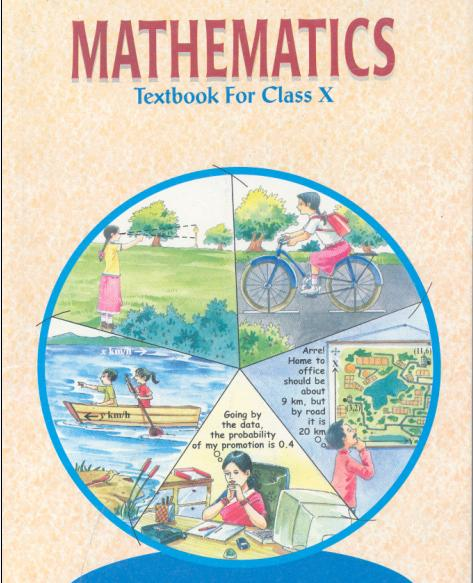## Class 10 Mathematics list of Chapters

1. Real Number
2. Polynomials
3. Pair of Linear Equations in Two Variables
5. Arithmetic Progressions
6. Triangles
7. Coordinate Geometry
8. Introduction to Trigonometry and its Applications
9. Circles
10. Constructions
11. Area Related to Circles
12. Surface Area and Volumes
13. Statistics and Probability

## Last Year Question Paper Class 10 Mathematics 2016

General Instructions:
1.
All questions are compulsory.
2. The question paper consists of 31 questions divided in to four sections A, B, C and D. Section-A comprises of 4 questions of 1 mark each; Section-B comprises of 6 questions of 2 marks each; Sections-C comprises of 10 questions of 3 marks each and Section -D comprises of 11 questions of 4 marks each.
3. There is no overall choice in this question paper.
4. Use of calculator is not permitted.

### Section-A

Question number 1 to 4 carry one mark each

1. In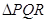,S and T are points in the sides PQ and PR respectively such that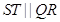. If PS = 4 cm, PQ = 9 cm and PR = 4.5 cm, then find PT.

2. Find the value of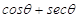, when it is given that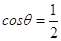3. If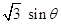, find the value of.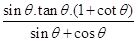4. Find the sum of upper limit and lower limit of the class interval in which the 20th observation of the following data lies:

 Class Interval 0-100 100-200 200-300 300-400 400-500 500-600 600-700 Frequency 5 7 6 3 20 4 8

### SECTION-B

Question number 5 to 10 carry two marks each.

5. Find the prime factorization of the denominator of the rational number equivalent to 8.39.

6. Show that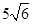is an irrational number

7. Find the quadratic polynomial whose zeroes are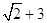and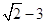.

8. State which of the two triangles given in the figure are similar. Also, state the similarity criterion used.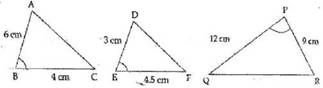9. Prove that: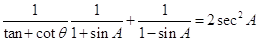10. Determine missing frequency x, from the following data, when Mode is 67.

 Class 40-50 50-60 60-70 70-80 80-90 Frequency 5 X 15 12 7

### SECTION C

Questions number 11 to 20 carry three marks each.

11. Use Euclid division lemma to show that square of any positive integer cannot be of the from 5m+2 or 5m +3 for some integer m.

12. A man has certain note of denomination 20 and 5 which amount to 380. If the number of notes of each kind are interchanged, they amount to 60 less than before. Find the number of notes of each denomination.

13. Divide the polynomial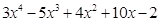by the polynomial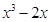and verify the division algorithm.

14. Show graphically the following pair of linear equations if inconsistent:
2x – 2y – 2 = 0
3x – 3y + 5 = 0

15.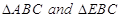are in the same base BC. If AE produced intersects BC at D then, prove that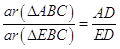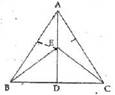16. In a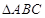, AD is perpendicular to BC and AD2 =BD x CD, Prove that ABC is a right-angled Triangle.

17.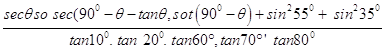18. Prove that:
(sin A + cosec A )2 + (cos A + sec A)2 = 7 + tan2 A + cot2 A

19. The following data gives the information on the observed lifetimes (in hours) of 150 electrical components:

 Lifetime (in hours) 0-20 20-40 40-60 60-80 80-100 Frequency 15 10 35 5 40

Find the mode of the distribution.
20. The weekly pocket money of the students of class ix of a school are given in the following table:

 Pocket money (in) 0-40 40-80 80-120 120-160 160-200 200-240 Number of students 5 7 15 10 5 8

Find the median for the above data.

### Section D

Question numbers 21 to 31 carry four marks each.

21. Can the number 6n,n being a natural number, end with the digit 5? Give reasons.

22. Draw the graph of the following pair of linear equations:
X + 3y = 6 and 2x – 3y = 12
Find the ratio of the areas of the two triangles formed by first line, x = 0, y = 0 and second line x = 0, y = 0

23. Obtain all other zeroes of the polynomial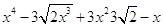if two of its zeroes are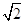and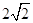.

24. Mr. Sharma and Mr. Arora are family friends and they decided to go for a trip with family. For the trio they reserved their rail tickets. Mr. Arora has not taken a half ticket for his child who is 6 years old where as Mr. Sharma has taken half tickets for his two children who are 65 years and 8 years old. A railway half ticket costs half of the full fare but the reservation charges are the same as in a full ticket. Mr. and Mrs. Arora paid 1700, while Mr. and Mrs. Sharma paid 2700. Find the full fare of one ticket and the reservation charges per ticket what difference you find in their behavior and which one you will choose for you self?

25. In the given figure, ABC is a triangle and GHED is a rectangle. BC = 12 cm, HE = 6cm, FC = BF and altitude AF = 24 cm. Find the area of the rectangle.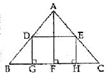26. “In a triangle if square of one side is equal to the sum of the squares of the other two sides, then the angle opposite the first side is a right angle’. Prove it.

27. If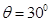, verify the following:
i)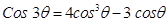,
ii)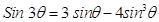28. Prove that: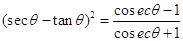29.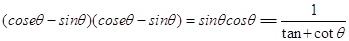30. The daily income of 150 families if given below. Calculate the arithmetic mean.

 Income No. of families More than75 150 More than85 140 More than95 115 More than105 95 More than115 70 More than125 60 More thanl35 40 More than145 25

31. The following table gives the daily income of 50 workers of a factory. draw both types (“less than type’ and ‘greater than type’) gives

 Daily income(in) 100-120 120-140 140-160 160-180 180-200 Number of workers 12 14 8 6 10s

These are questions only. To view and download complete question paper with solution install myCBSEguide App from google play store or login to our student dashboard.

## CBSE Question Paper 2016 class 10 Mathematics

Download class 10 Mathematics question paper with solution from best CBSE App the myCBSEguide. CBSE class 10 Mathematics paper 2016 in PDF format with solution will help you to understand the latest question paper pattern and marking scheme of the CBSE board examination. You will get to know the difficulty level of the question paper.

## Previous Year Question Paper for class 10 in PDF

question papers 2019, 2018, 2017, 2016, 2015, 2014, 2013, 2012, 2011, 2010, 2009, 2008, 2007, 2006, 2005 and so on for all the subjects are available under this download link. Practicing real question paper certainly helps students to get confidence and improve performance in weak areas.

To download CBSE Question Paper class 10 Mathematics, Science, Social Science, English Communicative, English Language and Literature, Hindi Course A, Hindi Course B, and Other subjects; do check myCBSEguide app or website. myCBSEguide provides sample papers with solution, test papers for chapter-wise practice, NCERT solutions, NCERT Exemplar solutions, quick revision notes for ready reference, CBSE guess papers and CBSE important question papers. Sample Paper all are made available through the best app for CBSE students and myCBSEguide website.Test Generator

Create question paper PDF and online tests with your own name & logo in minutes.myCBSEguide

Question Bank, Mock Tests, Exam Papers, NCERT Solutions, Sample Papers, Notes